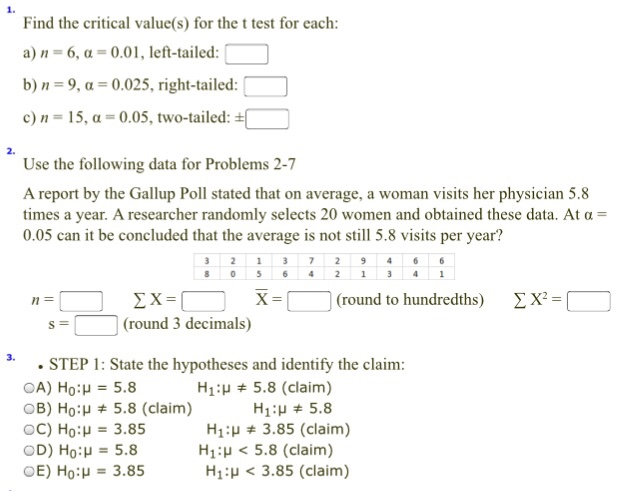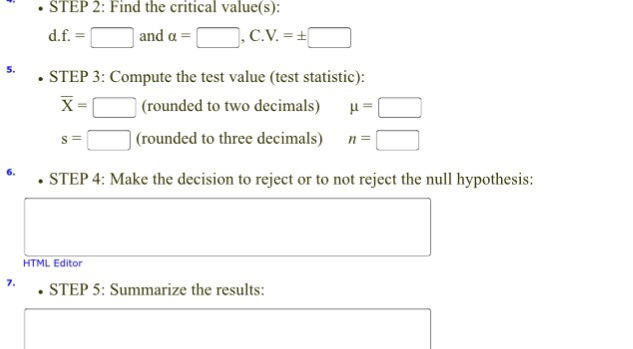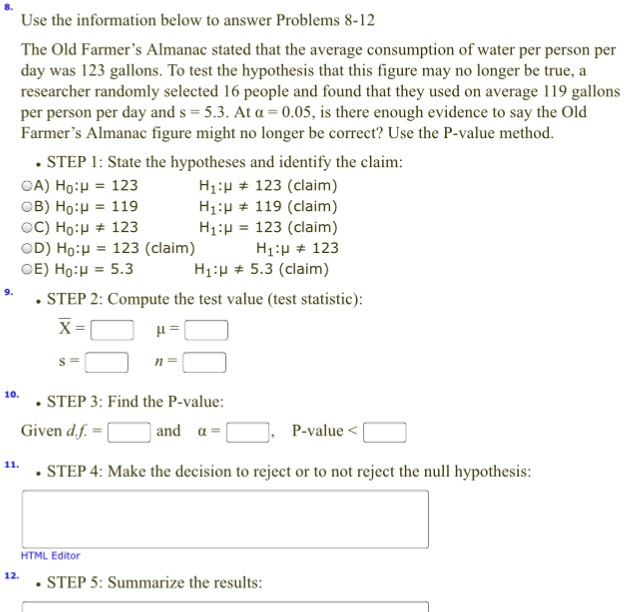# Find the critical value(s) for the t test for each

\$5.00Find the critical value(s) for the t test for each: n = 6, alpha = 0.01, left-tailed: n = 9, alpha= 0.025, right-tailed: n = 15, alpha = 0.05, two-tailed: Use the following data for Problems 2-7 A report by the Gallup Poll stated that on average, a woman visits her physician 5.8 times a year. A researcher randomly selects 20 women and obtained these data. At alpha = 0.05 can it be concluded that the average is not still 5.8 visits per year? State the hypotheses and identify the claim Find the critical value(s): Computer the test value (test statistic): Make the decision to reject or to not reject the null hypothesis: summarize the results: Use the information below to answer Problems 8-12 The Old Farmer’s Almanac stated that the average consumption of water per person per day was 123 gallon. To test the hypothesis that this figure may no longer be rue, a researcher randomly selected 16 people and found that they used on ovarage 119 gallons per person per day and s = 5.3 At alpha = 0.05, is there enough evidence to say the Old Farmer’s Almanac figure might no longer be correct? Use the P-value method.

SKU: find-the-critical-values-for-the-t-test-for-each Category: